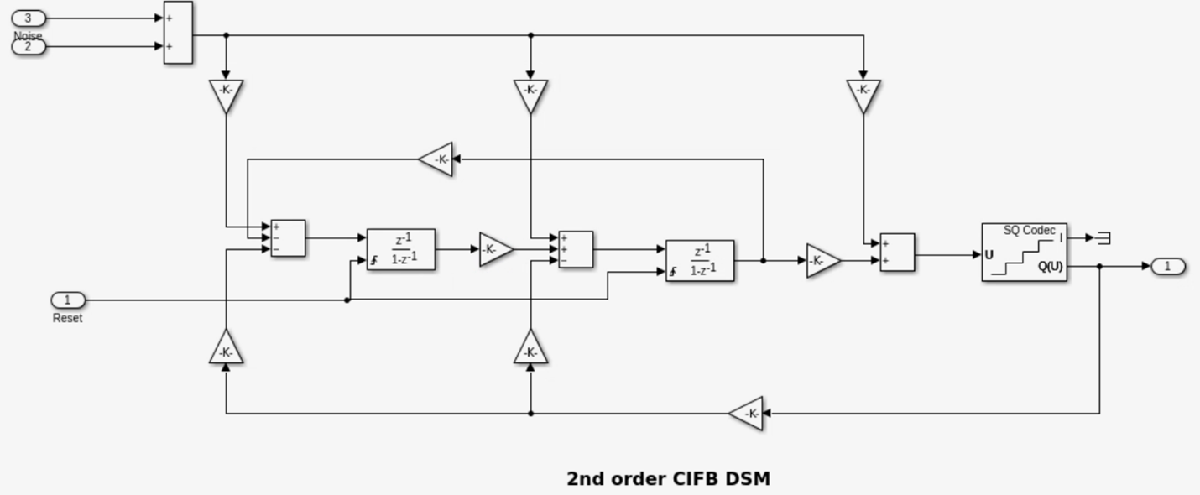# Delta Sigma Modulator Data Converter with Half-Band Filter for Decimation

This example shows how to use the Delta Sigma Modulator (DSM) data converter block with a downstream decimation filtering scheme. A half-band filter is used in this example for decimation.

DSMs are a type of oversampled data converters that convert analog input to a digital bit-stream output. The sampling rate used is usually in excess of the Nyquist rate. The output bit-stream data comes out at the higher sampling rate. The purpose of a decimation filter is to reduce the data rate to a normal range and extract information from the data stream.

### Design Flow

A typical design flow for modeling a DSM for a specific application is as follows: 1) Given a system specification, start with one particular architecture, for example 2nd order CIFB. Simulate the model and look at performance (Output spectrum, SNR etc) 2) For the same architecture, model with the Noise impairment to see how performance changes with impairment. 3) Try simulating the same architecture but a higher order (example 4th order CIFB) to see if better performance is achieved. 4) Calculate the switched capacitor values for architectures in step 1 and 3 and see if they can be practically implemented given circuit area specifications. 5) Try the above methods with a completely different architecture (example 2nd order CRFF), repeat steps 1-4

### Analog to digital conversion

Open the model DSM_Decimation_filter attached to this example:

```open_system('DSM_Decimation_Filter.slx'); ```An analog input (sine wave) stimulus is converted into a digital bit-stream. The low frequency signal is over-sampled and input to the DSM block. The DSM block converts the analog signal to a digital bit-stream. The specification for the DSM block is as follows:

1) Sampling Frequency = 50KHz 2) Bandwidth = 1KHz 3) Over Sampling Ratio = 25

The output of the DSM is seen using a spectrum analyzer which shows the signal profile coming out at a data rate of 50Ksps. The output of the DSM is made to go through a half-band filter and the 24-bit output of the filter can be seen on a scope. The spectrum of the decimated filter output coming out at the rate of 32sps can be seen on another spectrum analyzer.

### Delta sigma modulator

The DSM block is a masked subsystem containing variant subsystems to define different DSM architectures which can model 4 different architectures with orders ranging from 2 to 6. A total of 20 different architectures can be modeled. DSM architectures can be classified as:

1) CIFB: Cascade of Integrators, Feedback 2) CRFB: Cascade of Resonators, Feedback 3) CIFF: Cascade of Integrators, Feedforward 4) CRFF: Cascade of Resonators, Feedforward The user can choose the architecture in the mask for the DSM block.### Noise

The DSM block can model system noise such as kT/C which is modeled as an input referred noise source.The user can enable or disable the noise impairment by making use of the checkbox provided in the mask s Noise tab.There are entries for the system signal-to-noise ratio (SNR), input signal power and bandwidth. Default values specified in the mask will be used in this example.

### Switched capacitor calculation

The DSM block can calculate the values of the switched capacitors needed to implement the discrete time DSM being modeled. This calculation uses technology specific parameters from a CMOS process development kit (PDK) and produces values for unit capacitance, sampling and integrating capacitances to be used in the circuit. These values can be used directly in the circuit schematic or layoutThe default PDK parameters are from a 90nm CMOS process node.

### Half-band filter

Half-band filter is a low pass filter which reduces the maximum bandwidth of sampled data by one octave. It is a topology used in downstream decimation of the DSM output. This example contains a half band filter with coefficients.### DSM output

Spectrum analyzer output (50Ksps) from a 2nd order CIFB without noise impairmentSpectrum analyzer output (50Ksps) from a 2nd order CIFB with noise impairmentSpectrum analyzer output from the half band filter (32sps)24 bit DSM output from a 2nd order CIFB DSMSwitched capacitor values for 2nd order CIFB DSM